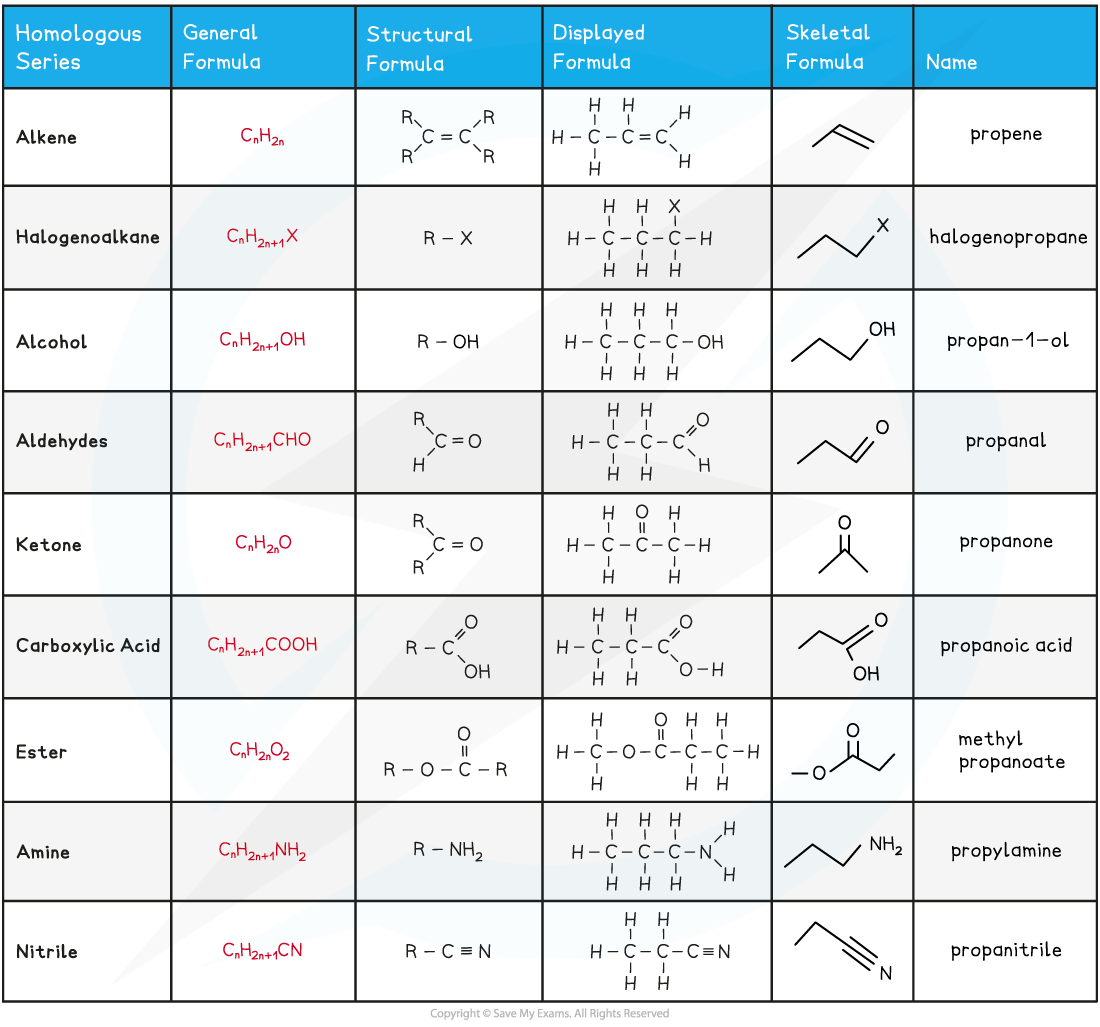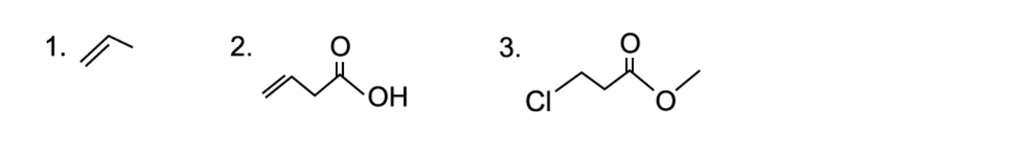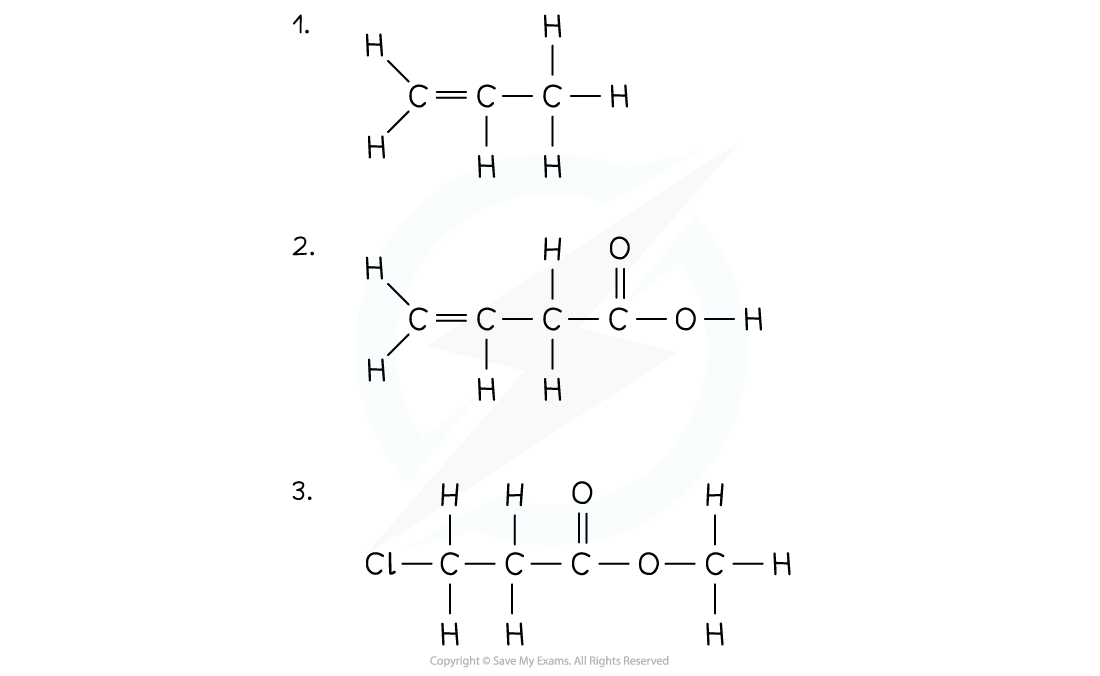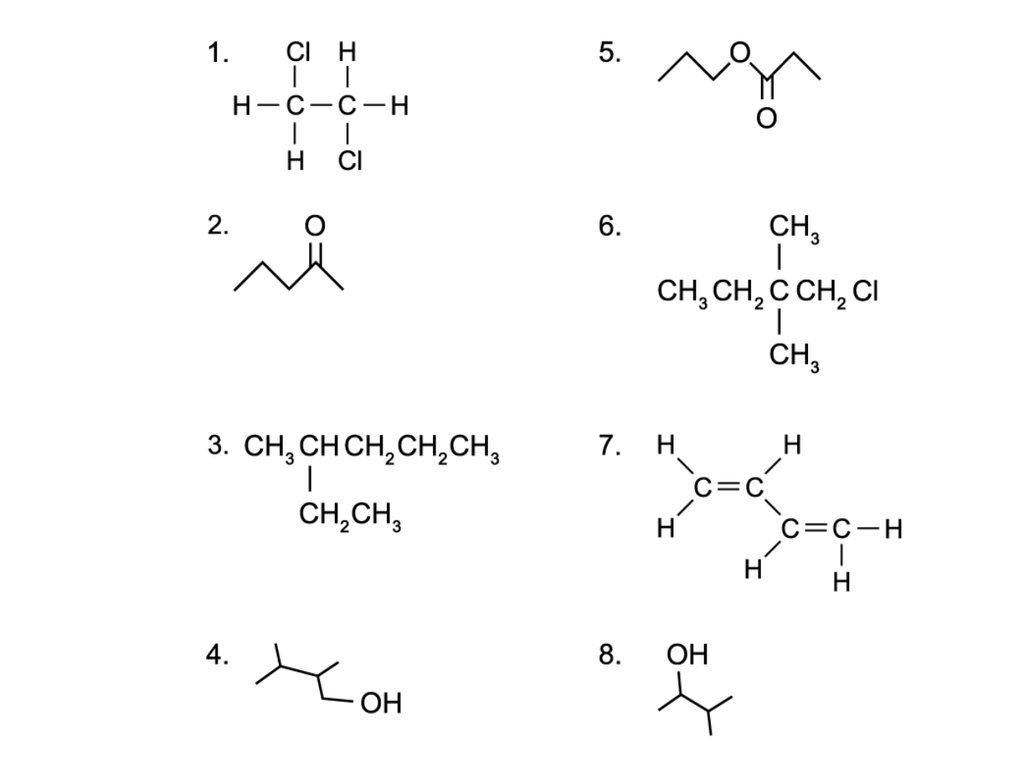# AQA A Level Chemistry复习笔记3.1.2 Types of Formulae

### Types of Formulae

• The general formula is a formula that represents a homologous series of compounds using letters and numbers
• Eg. the general formula of alkanes is CnH2n+2
• A homologous series is a group of organic compounds that have the same functional group, the same general formula and the same chemical properties

• The structural formula is a formula that shows how the atoms are bonded to each carbon atom in a molecule
• The displayed formula is a 2D representation of an organic molecule showing all its atoms (by their symbols) and their bonds (by single, double or triple bonds)
• The skeletal formula is a simplified displayed formula with all the carbon and hydrogen (C-H) bonds removed

Overview of the Formulae of Organic Compounds Table#### Worked Example

Drawing skeletal formulae of molecules

Draw the skeletal formula of the following molecules:

1. CH3(CH2)3OH
2. (CH3)2CHCH2OH
3. CH3CH2OCH2CH3#### Worked Example

Drawing displayed formulae of molecules

Draw the displayed formula of the following molecules:### Molecular & Empirical Formulae

• The molecular formula shows the number and type of each atom in a molecule
• Eg. the molecular formula of ethanoic acid is C2H4O2

• The empirical formula shows the simplest whole number ratio of the elements present in one molecule of the compound
• Eg. the empirical formula of ethanoic acid is CH2O

#### Worked Example

Deducing molecular & empirical formulaeDeduce the molecular and empirical formula of the following compounds: